Moisture content calculations

Calculating moisture content from wet weight and dry weight

Moisture Content of grain is usually determined on wet basis (wb), scientists sometimes use dry basis (db):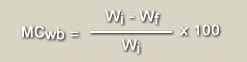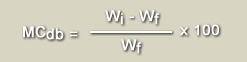MCwb = Moisture content wet basis [%]

MCdb = Moisture content dry basis [%]

Wi = Initial weight

Wf = Final weight

Conversion from MCwb to MCdb and back

Sometimes it is needed go convert from moisture content dry basis to moisture content wet basis.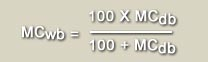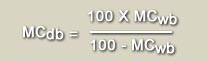Weight loss during drying

During drying, paddy grain will loose weight due to loss of moisture: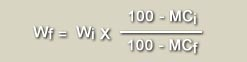Wi = Initial weight [g]

Wf = Final weight [g]

Example: 1000 kg of paddy is harvested at 25% MC, and dried down to 14% MC, what is the final weight of the dried grain?

Final weight of grain = 1000* (100-25)/(100-14) = 872 kg of paddy at 14 % MC